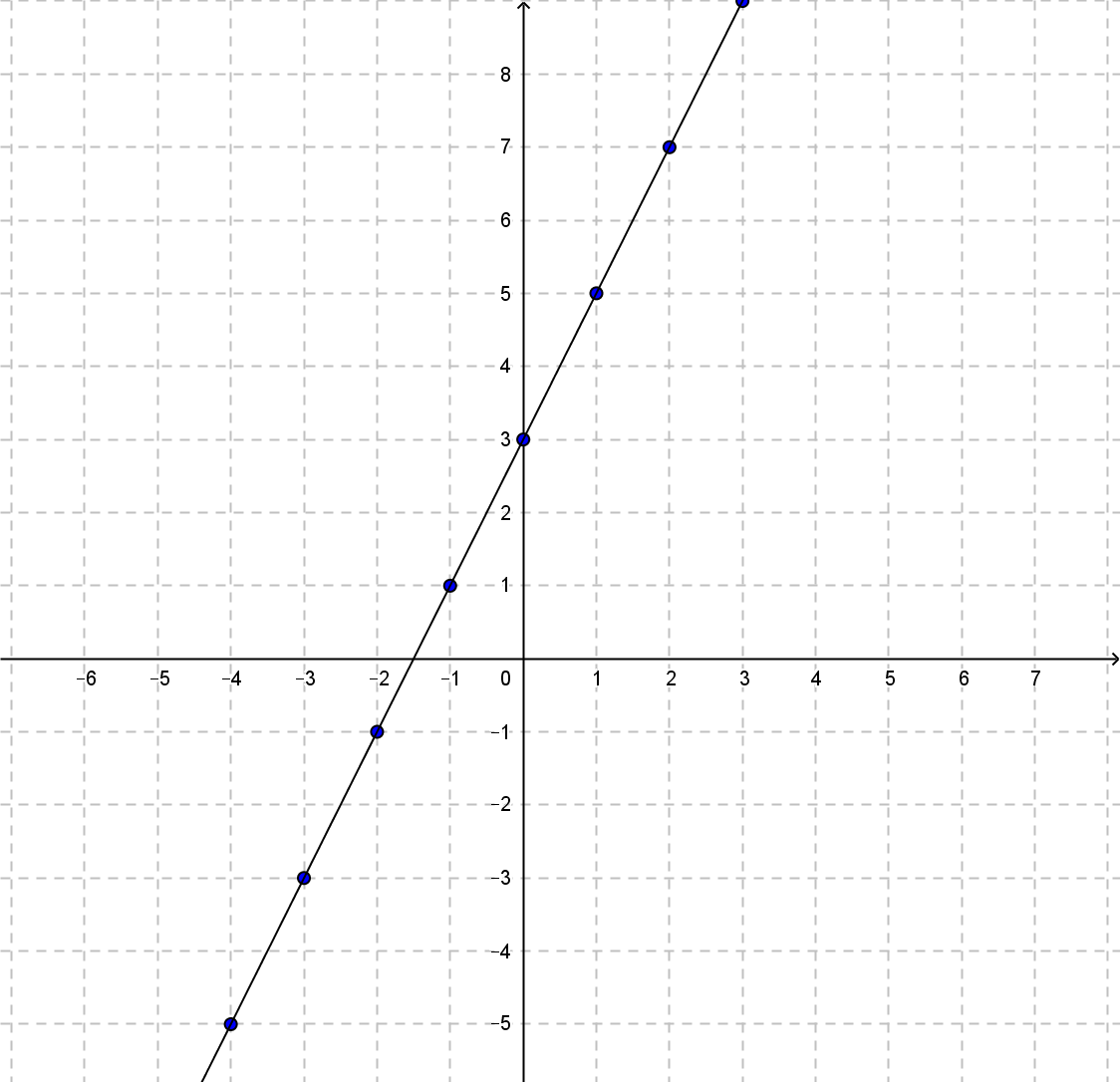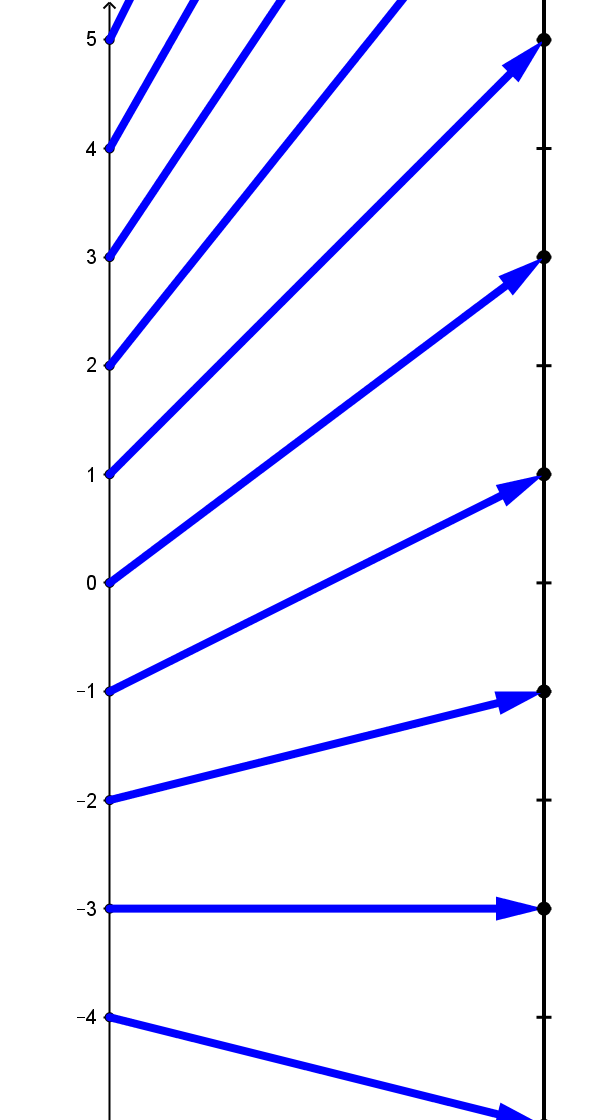##### Example TMD.0:  Suppose \$y\$ is a function of \$x\$ given by the equation \$y = f (x) = 2x+ 3\$.

 \$x\$ \$f(x)=2x+3\$ 5 13 4 11 3 9 2 7 1 5 0 3 -1 1 -2 -1 -3 -3 -4 -5 -5 -7
Table TMD1Graph TMD1Diagram TMD1

Table TMD1 shows a selection of the values this function relates.
The same information is visualized in Graph TMD1 and Diagram TMD1.

In Graph TMD1, the graph of the table values of the function \$f\$, we identify each pair of numbers \$a\$ and \$f(a)\$ from the table with the point in the plane with coordinates \$(a,f(a))\$.

In Diagram TMD1, the mapping diagram of the table values of the function \$f\$, we identify a point on the source line (on the left) with a number in the \$x\$ column on the table, \$a\$. We identify a point on the target line (on the right) with value of the function \$f\$ applied to that number, \$f(a)\$ also found in the table next to the number \$a\$. An arrow is drawn from the point on the source line, \$a\$, to the corresponding point on the target line, \$f(a)\$, that visualizes the relation between the corresponding numbers in the table.

For example, consider the pair from Table TMD1, \$1\$ and \$5\$. In Graph TMD1 this pair corresponds to the point in the graph with coordinates \$(1,5)\$. In  mapping Diagram TMD1 this pair corresponds to the point \$1\$ on the left line of the diagram, the point \$5\$ on the right line the diagram and the arrow from the points representing \$1\$ to \$5\$ indicating the function relating the two numbers.

A mapping diagram visualizes a corresponding function table. The numbers in one row of the table are represented by points on the two lines in the figure. The function relation that the table displays implicitly by having corresponding numbers in the same row is visualized in the mapping diagram by the arrow.  While the relative size of the numbers in the target column of the table is not represented in the display, the mapping diagram uses the number line order to represent this aspect of the function's values.